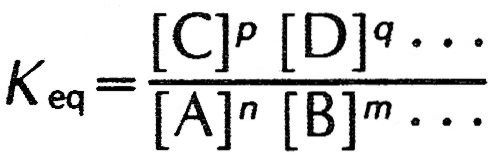Summary Article: chemical equilibrium
From The Columbia Encyclopedia

state of balance in which two opposing reversible chemical reactions proceed at constant equal rates with no net change in the system. For example, when hydrogen gas, H2, and iodine gas, I2, are mixed, and gaseous hydrogen iodide, HI, is formed according to the equation H2 + I2 → 2HI, no matter how long the reaction is allowed to proceed some quantity of hydrogen and iodine will remain unreacted. The reason reactants in a reversible reaction are never completely converted to product is that an opposing reaction is taking place simultaneously, i.e., some of the newly formed HI is being converted back into hydrogen and iodine. For any particular temperature, a point of equilibrium is reached at which the rates of the two opposing reactions are equal and there is no further change in the system. This equilibrium point is characterized by specific relative concentrations of reactants and products and will also be reached from the opposite direction, i.e., if one starts with hydrogen iodide and allows it to decompose into hydrogen and iodine. The equilibrium point can be described by the mass action expression, which defines the equilibrium constant, Keq, in terms of the ratio of the molar concentrations of the products to those of the reactants. For the reversible reaction used as an example, the equilibrium constant is Keq=[HI]2/[H2][I2]; for the general reversible reaction nA + mB + · · · ⇋ pC + qD + · · ·, the equilibrium constant is:where [A], [B], [C], [D], … are the molar concentrations of the substances and n, m, p, q, … are the coefficients of the balanced chemical equation. The larger the equilibrium constant for a given reaction, the more the reaction is favored, since a larger value of Keq means larger concentrations of the products relative to the reactants. The equilibrium constant is related to the change in the standard free energy, , of the system by the equation Δ = −RT. ln Keq, where R is a constant, T is the temperature in degrees Kelvin, and ln Keq is the natural logarithm of the equilibrium constant. Chemical equilibrium can be defined for many types of chemical processes, such as dissociation of a weak acid in solution, solubility of slightly soluble salts, and oxidation-reduction reactions. In all of these cases, the equilibrium constant or its analogue is defined for certain conditions of temperature and other factors. If any of these factors change, the system will respond to establish a new equilibrium, in accordance with Le Châtelier's principle.The Columbia Encyclopedia, © Columbia University Press 2018

### Related Articles

##### Full text Article chemical equilibrium
Philip's Encyclopedia

Balance in a reversible reaction , when two opposing reactions proceed at constant equal rates with no net change in the system. The initial...

See more from Credo